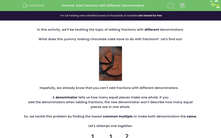# Add Fractions with Different Denominators

In this worksheet, students will practice adding fractions with different denominators by finding the lowest common multiple.Key stage:  KS 2

Curriculum topic:   Number: Fractions, Decimals and Percentages

Popular topics:   Fractions worksheets

Difficulty level:#### Worksheet Overview

In this activity, we'll be tackling the topic of adding fractions with different denominators.

What does this yummy looking chocolate cake have to do with fractions?  Let's find out!Hopefully, we already know that you can't add fractions with different denominators.

denominator tells us how many equal pieces make one whole. If you add the denominators when adding fractions, the new denominator won't describe how many equal pieces are in one whole.

So, we tackle this problem by finding the lowest common multiple to make both denominators the same.

Let's attempt one together:First, we look at the different denominators and we must find the lowest common multiple of  2 and  i.e.

1 × 2 = 2

2 × 2 = 4

3 × 2 = 6

1 × 3 = 3

2 × 3 = 6

So, we've found our lowest common multiple is 6.

Now, we need to make both our denominators 6.  The key rule to remember here is that whatever you do to the denominator, you must also do to the numerator.So, if we apply the sums above we end up with:Pretty simple right?

Now it's over to you. You can always return to this explanation if you get stuck, by clicking on the pink button at the side of the screen.

Good luck!

### What is EdPlace?

We're your National Curriculum aligned online education content provider helping each child succeed in English, maths and science from year 1 to GCSE. With an EdPlace account you’ll be able to track and measure progress, helping each child achieve their best. We build confidence and attainment by personalising each child’s learning at a level that suits them.

Get started